# Checking Rank Estimates

In :
import numpy as np
import numpy.linalg as la
import matplotlib.pyplot as pt


Let's make two particle collections: sources and targets

In :
sources = np.random.randn(2, 200)
targets = np.random.randn(2, 200) + 10

pt.plot(sources, sources, "go")
pt.plot(targets, targets, "ro")

Out:
[<matplotlib.lines.Line2D at 0x7fec117b6f98>]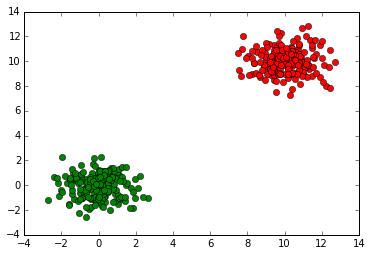What's our convergence ratio $\rho$: $$\rho=\frac{d(c,\text{furthest target})}{d(c,\text{closest source})}?$$

Well, what should $c$ be, really? (Technically, we can use any $c$, so we're free to choose whichever we think is best.)

In :
c = np.sum(targets, axis=1) / targets.shape
c

Out:
array([ 10.05952737,  10.01047655])

In :
pt.plot(sources, sources, "go")
pt.plot(targets, targets, "ro")
pt.plot(c, c, "bo")

Out:
[<matplotlib.lines.Line2D at 0x7fec114614a8>]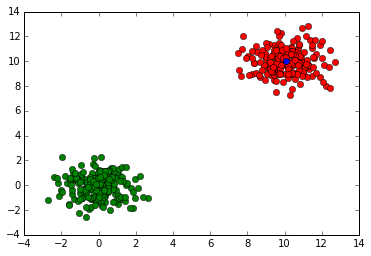## Assemble the interaction matrix

In :
all_distvecs = sources.reshape(2, 1, -1) - targets.reshape(2, -1, 1)
dists = np.sqrt(np.sum(all_distvecs**2, axis=0))
interaction_mat = np.log(dists)


## Estimate the rank depending on precision $\varepsilon$

First, obtain an idea of what $\rho$ is:

In :
dist_tgt_to_c = np.sqrt(np.sum((c.reshape(2, 1) - targets)**2, axis=0))
dist_src_to_c = np.sqrt(np.sum((c.reshape(2, 1) - sources)**2, axis=0))

rho = np.max(dist_tgt_to_c) / np.min(dist_src_to_c)
rho

Out:
0.26574750307862688


Then plot the numerical rank depending on epsilon:

In :
_, sigma, V = la.svd(interaction_mat)
pt.semilogy(sigma)

Out:
[<matplotlib.lines.Line2D at 0x7fec10f34470>]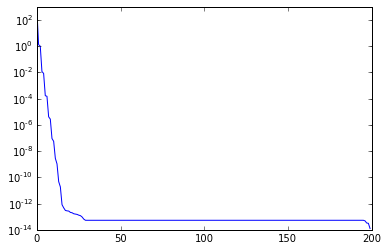In :
eps_values = 10**(-np.linspace(1, 12))


For the given precisions $\varepsilon$, find the associated numerical rank:

In :
def numrank(eps):
return np.sum(sigma > eps)

ranks = [numrank(e) for e in eps_values]

pt.semilogx(eps_values, ranks)

Out:
[<matplotlib.lines.Line2D at 0x7fec10ab2240>]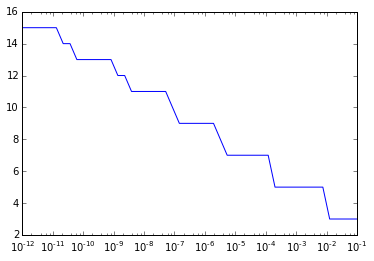Now compare with our estimate:

In :
pt.semilogx(eps_values, ranks)
pt.semilogx(eps_values, (np.log(eps_values)/np.log(rho)-1)**2)

Out:
[<matplotlib.lines.Line2D at 0x7fec10d7d128>]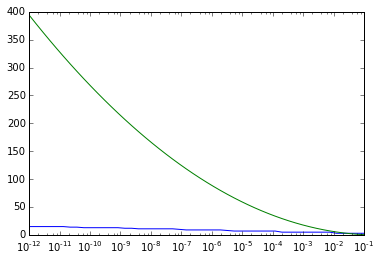• We estimated that the rank would grow quadratically.
• Comments on how good our estimate is?